# GRE Subject Test: Math : Applications of Derivatives

## Example Questions

2 Next →

### Example Question #1 : Taylor Series

Write out the first four terms of the Taylor series about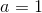for the following function: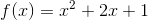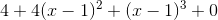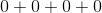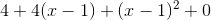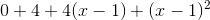Explanation:

The Taylor series about x=a of any function is given by the following: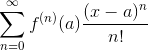So, we must find the zeroth, first, second, and third derivatives of the function (for n=0, 1, 2, and 3 which makes the first four terms):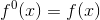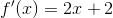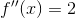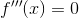The derivatives were found using the following rule: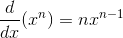Now, evaluated at x=a=1, and plugging in the correct n where appropriate, we get the following: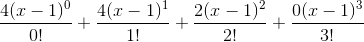which when simplified is equal to.

### Example Question #2 : Taylor's Theorem

Find the first two terms of the Taylor series about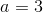for the following function: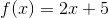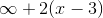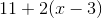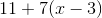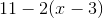Explanation:

The general formula for the Taylor series about x=a for a function isFirst, we must find the zeroth and first derivative of the function.

The zeroth derivative of a function is just the function itself, so we only have to find the first derivative: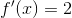The derivative was found using the following rule:Now, write the first two terms of the sequence (n=0 and n=1):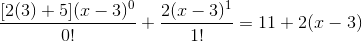### Example Question #11 : Applications Of Derivatives

Write out the first three terms of the Taylor series for the following function about: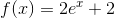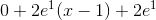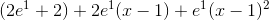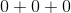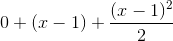Explanation:

The general formula for the Taylor series of a given function about x=a is.

We were asked to find the first three terms, which correspond to n=0, 1, and 2. So first, we need to find the zeroth, first, and second derivative of the given function. The zeroth derivative is just the function itself.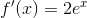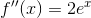The derivatives were found using the following rules: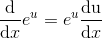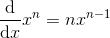Now use the above formula to write out the first three terms: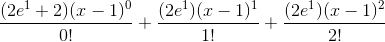Simplified, this becomes2 Next →

### All GRE Subject Test: Math Resources